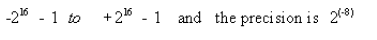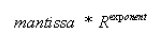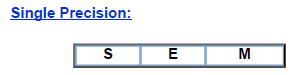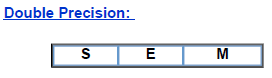Courses

# Representation of Real Number Computer Science Engineering (CSE) Notes | EduRev

## Computer Science Engineering (CSE) : Representation of Real Number Computer Science Engineering (CSE) Notes | EduRev

The document Representation of Real Number Computer Science Engineering (CSE) Notes | EduRev is a part of Computer Science Engineering (CSE) category.
All you need of Computer Science Engineering (CSE) at this link: Computer Science Engineering (CSE)

Representation of Real Number:
Binary representation of 41.6875 is 101001.1011

Therefore any real number can be converted to binary number system

There are two schemes to represent real number :

• Fixed-point representation
• Floating-point representation

Fixed-point representation:

Binary representation of 41.6875 is 101001.1011

To store this number, we have to store two information,
--    the  part  before decimal point and
--    the  part  after decimal point. This is known as fixed-point representation where the position of decimal point is fixed and number of bits before and after decimal point are also predefined.

If we use 16 bits before decimal point and 8 bits after decimal point, in signed magnitude form, the range isOne bit is required for sign information, so the total size of the number is 25 bits

(  1(sign)  +  16(before decimal point)  +  8(after decimal point)  ).

Floating-point representation:
In this representation, numbers are represented by a mantissa comprising the significant digits and an exponent part of Radix R.    The format is:Numbers are often normalized, such that the decimal point is placed to the right of the  first non zero digit.
For example, the decimal number,

5236     is   equivqlent to 5.236 * 103

To store this number in floating point representation, we store 5236 in mantissa part and 3 in exponent part.

IEEE standard floating point format:
IEEE has proposed two standard for representing floating-point number:

• Single precision
• Double precisionS: sign bit: 0 denoted + and 1 denotes -E: 8-bit excess -27 exponentM: 23-bit mantissa S: sign bit: 0 denoted + and 1 denotes -E: 11-bit excess -1023 exponentM: 52-bit mantissa
Offer running on EduRev: Apply code STAYHOME200 to get INR 200 off on our premium plan EduRev Infinity!

,

,

,

,

,

,

,

,

,

,

,

,

,

,

,

,

,

,

,

,

,

;